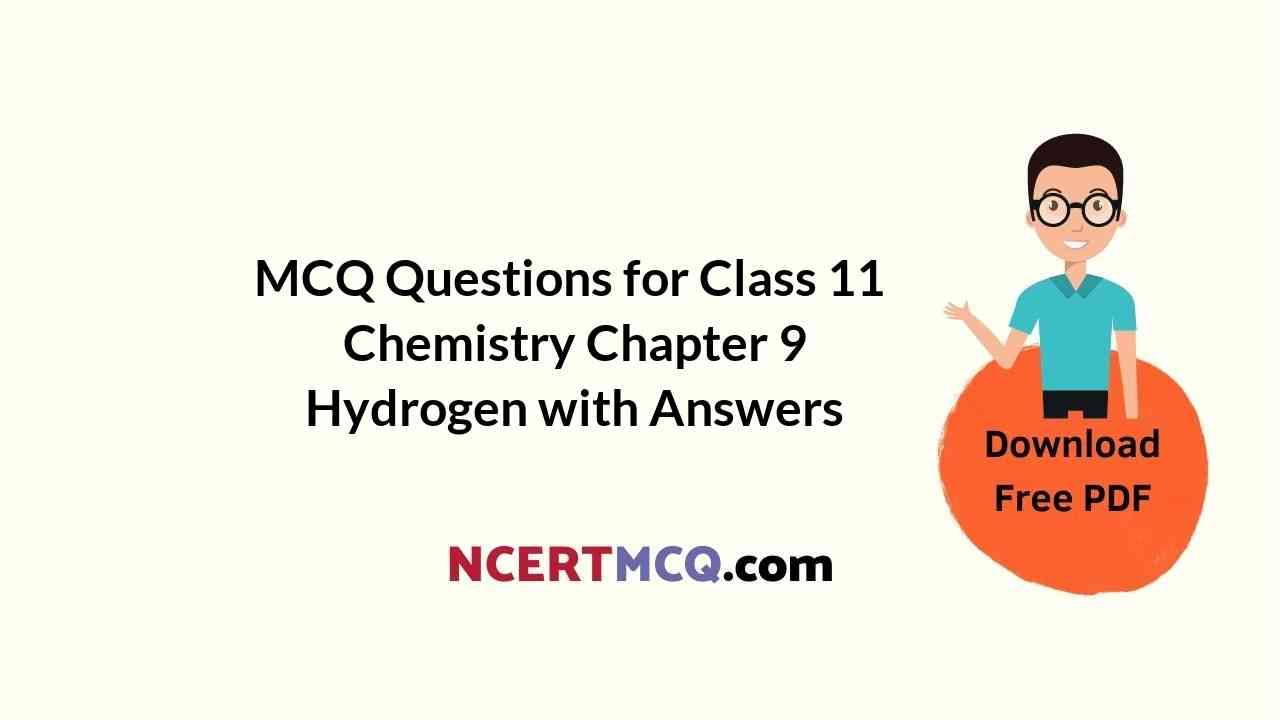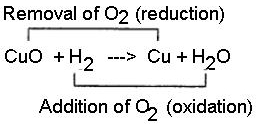Check the below NCERT MCQ Questions for Class 11 Chemistry Chapter 9 Hydrogen with Answers Pdf free download. MCQ Questions for Class 11 Chemistry with Answers were prepared based on the latest exam pattern. We have provided Hydrogen Class 11 Chemistry MCQs Questions with Answers to help students understand the concept very well.

## Hydrogen Class 11 MCQs Questions with Answers

Hydrogen Class 11 MCQ Question 1.
Pure H2O2 is :
(a) Semi – solid
(b) Liquid
(c) Solid
(d) Gas

Explanation:
Hydrogen peroxide is the simplest kind of peroxide available (oxygen-oxygen single bond). It is a colourless liquid and is used in aqueous solution for safety reasons. It acts as a bleaching agent and is also used as a disinfectant. Concentrated hydrogen peroxide is a very reactive oxygen species and is used as a propellant in rocketry. The chemical formula for hydrogen peroxide is H2O2.

MCQ On Hydrogen Class 11 Question 2.
The freezing point of heavy water is
(a) 0°C
(b) 3.8°C
(c) 4°C
(d) 1°C

Hydrogen MCQ Class 11 Question 3.
H2O2 used in rocket has the concentration:
(a) 50%
(b) 90%
(c) 70%
(d) 30%

Explanation:
H2O2 is used as an oxidant for rocket fuel. Usually, 90% concentration of H2O2 is used.

MCQ Of Hydrogen Class 11 Question 4.
Which of the following hydrides are generally nonstochiometric in nature?
(a) Ionic Hydrides
(b) Molecular Hydrides
(c) Interstitial Hydrides
(d) All of the Above.

Explanation:
Interstitial hydrides are non- stoichiometric hydrides and thus deficit in hydrogen. Transition and inner transition elements at relevant temperature absorb hydrogen into the interstices of their lattices to yield metal like hydrides.

Class 11 Hydrogen MCQ Question 5.
What is the product of the reaction of H2O2 with Cl2?
(a) O2 + HOCl
(b) HCl + O2
(c) H2O + HCl
(d) HCl + H2

Explanation:
H2O2 + Cl2 → 2HCl + O2

Class 11 Chemistry Hydrogen MCQ Question 6.
Water shows anomalous behavior between
(a) 0 to 4°C
(b) 0 to 5°C
(c) 0 to -4°C
(d) 4 to 0°C

Explanation:
Water show a wide range of anomalies compared to similar liquids or hydrides of other group 16 elements. One of the most talked-about anomalies is the formation of ice from liquid water and how the density changes when we cool the water.

O is a small atom and highly electronegative compared to Hydrogen. So O attracts the covalent clouds of O-H towards itself, thus making water a polar molecule. The hydrogen bonding in water molecules causes high boiling point and liquid state compared to other hydrides of group 16.

When we cool water from higher temperature, the density steadily decreases. At 4 degree C it is the highest. But below 4 degree C, The H-bonds break between the molecules and the molecules get drifted further apart, the volume increases and density decreases. The crystalline form of water is ice. At atmospheric pressure ice crystallises in the hexagonal form, but at very low temperatures it condenses to cubic form leaving gap between the structures, thus increasing the volume again.

Density of ice is less than that of water. Therefore, an ice cube floats on water. In winter season ice formed on the surface of a lake provides thermal insulation which ensures the survival of the aquatic life.

Class 11 Chemistry Chapter 9 MCQ Question 7.
Which of the following statements regarding hydrogen peroxide is/ are incorrect?
(a) As aerating agent in production of sponge rubber
(b) As an antichlor
(c) For restoring white colour of blackened lead painting
(d) All of the above

Answer: (d) All of the above
Explanation:
H2O2 show all these properties

Hydrogen Chapter Class 11 MCQ Question 8.
Atomic hydrogen is called
(a) Protium
(b) Deutrium
(c) Nascent Hydrogen
(d) Tritium

Explanation:
A hydrogen atom is an atom of the chemical element hydrogen. The electrically neutral atom contains a single positively charged proton and a single negatively charged electron bound to the nucleus by the Coulomb force. Atomic hydrogen constitutes about 75% of the baryonic mass of the universe.

In everyday life on Earth, isolated hydrogen atoms (called “atomic hydrogen”) are extremely rare. Instead, a hydrogen atom tends to combine with other atoms in compounds, or with another hydrogen atom to form ordinary (diatomic) hydrogen gas, H2. “Atomic hydrogen” and “hydrogen atom”

Hydrogen MCQs With Answers Question 9.
The volume strength of 1.5 NH2O2 solution is :
(a) 4.8
(b) 5.2
(c) 8.8
(d) 8.4

Explanation:
Strength =Normality ×Equivalent Weight of H2O2
= 1.5N × 1.7gL-1 = 25.5gL-1
2H2O2 → 2H2O + O2
68g 22400 mLat STP
68 g of H2O gives = 22400 mL of O2 at STP
25.5 gH2O2 gives = 2240068 × 25.5 = 8400 mL of O2 at STP
25.5g of H2O2 is present in 1000 mL of H2O2 solution
1000 mL of H2O2 gives 8400 mL of O2 at STP
1 mL of H2O2 gives 84001000 mL of O2 at STP
= 8.4mL of O2
Hence, volume strength of 1.5NH2O2 = 8.4 volume.
Or mass of H2O2 in 1.5N solution
= Equivalent Weight of H2O2 × 1.5N
= 17 × 1.5 = 25.5 g/L
Hence, volume strength of 1.5NH2O2 solution
= 22.4 × 25.568 = 8.4

Hydrogen Class 11 MCQ Pdf Question 10.
Water gas is mixture of hydrogen H2 and
(a) CO
(b) CO2
(c) Cl2
(d) SO2

Explanation:
Water gas is a combustion fuel containing carbon monoxide (CO) and hydrogen gas (H2). Water gas is made by passing steam over heated hydrocarbons.

MCQ Questions For Class 11 Chemistry Chapter 9 Question 11.
Hydrogen has isotopes
(a) 2
(b) 3
(c) 4
(d) 5

Explanation:
Hydrogen has three main isotopes; Protium (1H), deuterium (²H) and tritium (³H). These isotopes form naturally in nature. Protium and deuterium are stable. Tritium is radioactive and has a half-life of about 12 years. Scientists have created four other hydrogen isotopes (4H to 7H), but these isotopes are very unstable and do not exist naturally.
The main isotopes of hydrogen are unique because they are the only isotopes that have a name.

Hydrogen MCQs Class 11 Question 12.
The freezing point of heavy water is
(a) 0°C
(b) 3.8°C
(c) 4°C
(d) 1°C

Class 11 Chemistry Chapter 9 MCQ With Answers Question 13.
Pure H2O2 is:
(a) Semi – solid
(b) Liquid
(c) Solid
(d) Gas

Explanation:
Hydrogen peroxide is the simplest kind of peroxide available (oxygen-oxygen single bond). It is a colourless liquid and is used in aqueous solution for safety reasons. It acts as a bleaching agent and is also used as a disinfectant. Concentrated hydrogen peroxide is a very reactive oxygen species and is used as a propellant in rocketry. The chemical formula for hydrogen peroxide is H2O2.

MCQs On Hydrogen Class 11 Question 14.
_________________ on water decolourises H2O2
(a) O3
(b) Acidic KMnO4 solution
(c) Black Suspension of Lead Sulphide(PbS)
(d) None of these.

Explanation:
Lead sulphide (black suspension) on reaction with hydrogen peroxide forms lead sulphate and water. Lead sulphate as well as water are colourless, therefore lead sulphite decolourises on addition of hydrogen peroxide.
PbS(s) + 4H2O2 (aq) → PbSO4 (s) + 4H2O (aq)

MCQ Questions On Hydrogen Class 11 Question 15.
What is gram equivalent weight of hydrogen peroxide as reductant?
(a) 100
(b) 125
(c) 152
(d) None of the Above

Explanation:
As reductant hydrogen peroxide oxidises to oxygen. H2O2 → O2
​The change in oxidation number is 2
The molar mass = 34 g
Gram equivalent weight of H2O2 = ($$\frac{34}{2}$$)
​= 17 g
Equivalent weight = 152

Question 16.
Hydrogen is the most abundant element on earth after______.
(a) Oxygen
(b) Carbon
(c) Sulphur
(d) None of the Above

Explanation:
The mass-abundance of the nine most abundant elements in the Earths crust is approximately: oxygen 46%, silicon 28%, aluminium 8.2%, iron 5.6%, calcium 4.2%, sodium 2.5%, magnesium 2.4%, potassium, 2.0%, and titanium 0 .61%.
Hydrogen is 0.14% on earths crust and other elements occur at less than 0.15%.

Question 17.
Atomic hydrogen is called
(a) Protium
(b) Deutrium
(c) Nascent Hydrogen
(d) Tritium

Explanation:
A hydrogen atom is an atom of the chemical element hydrogen. The electrically neutral atom contains a single positively charged proton and a single negatively charged electron bound to the nucleus by the Coulomb force. Atomic hydrogen constitutes about 75% of the baryonic mass of the universe.

In everyday life on Earth, isolated hydrogen atoms (called “atomic hydrogen”) are extremely rare. Instead, a hydrogen atom tends to combine with other atoms in compounds, or with another hydrogen atom to form ordinary (diatomic) hydrogen gas, H2. “Atomic hydrogen” and “hydrogen atom”

Question 18.
Hydrogen is a good __________ agent.
(a) Oxidizing
(b) Reducing
(c) Acidic
(d) Basic

Explanation:
Hydrogen acts as a good reducing agent means, when hydrogen gas is passed over hot metallic oxides of copper, lead, iron, etc. it removes oxygen from them and thus reduces them to their corresponding metal. Let us consider the following example, in each of which metallic oxide react with hydrogen. Metallic oxide act as oxidizing agents and hydrogen acts as a reducing agent.Question 19.
The volume of 10 volume H2O2 required to liberate 500 ml of O2 at S.T.P. is :
(a) 25 ml
(b) 50 ml
(c) 100 ml
(d) 125 ml

Explanation:
As 10 ml of oxygen is obtained at STP from H2O2 = 1 ml
Therefore, 500 ml of O2 is obtained at STP = 50 ml

Question 20.
Hydrogen is most __________ element in the universe.
(a) Abundant
(b) None
(c) Both
(d) Consumer